# What is Millman’s Theorem? Millman’s Theorem Formula

The network or circuit theorems are a tool to solve complex electrical problems. We use Millman’s Theorem to solve complex electrical circuits having many voltage or current sources. According to Millman’s theorem statement, the voltage and current sources can be replaced with a single current source with its equivalent resistance. We also use the following circuit theorems for solving network problems.

1. Kirchhoff’s current and voltage law
2. Thevenin’s theorem
3. Norton’s theorem
4. Superposition theorem

All the circuit theorems are equally important. However, we need to apply the network theorems according to the circuit diagram for a quick solution.

When the many practical voltages and current sources with their internal resistance are connected in the parallel circuit, then it becomes rather difficult to find the solution through the Norton or Thevenin theorem. However, we can easily find the solution of such a complex electrical circuit through Millman’s theorem.

We use Millman’s theorem to determine the voltage at the ends of the circuit which has many parallel circuits. With the use of Millman’s theorem, it is easy to compute the voltage at the end of the circuit for the 10 numbers or even more numbers of voltage generators connected in parallel. Millman’s theorem is also called the parallel generator theorem. Let us take a circuit diagram for a better understanding of Millman’s theorem.

Let there are three voltage sources V1, V2, and V3  connected in parallel and their internal resistances are R1, R2, and R3 respectively.## Millman’s Theorem Statement

Millman’s Theorem states that it is possible to replace numbers of voltage sources with finite internal resistance operating in parallel with a single voltage source with a series equivalent resistance. When the number of the independent voltage sources (V1, V2, V3, V4.—–Vn ) with internal resistance (R1, R2, R3, R4.—–Rn ) respectively, then this type of circuit can be replaced by equivalent voltage source V in the series with equivalent series resistance R. In the below-given circuit, we convert the voltage sources into the equivalent current sources.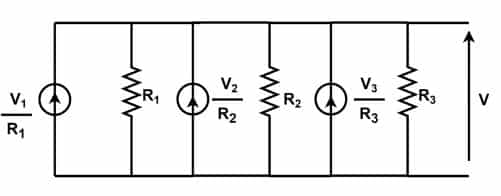## Millman’s Theorem Formula

According to Ohm’s law;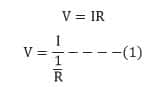Where I and R is the total circuit current and total circuit resistance respectively.

Total circuit Current is;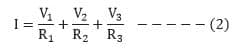Total circuit resistance is;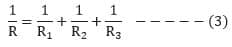Putting the values of equations (2) & (3) in equation (1), we get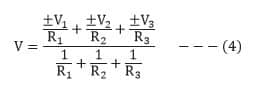The generalized form of Millman’s equation for “k” parallel circuits is;### Steps for Solving Millman’s Theorem

1. Change all the voltage sources of the circuit into current sources by dividing the potential of the sources with their internal resistance.Where- G is the conductance of the circuit.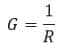2. Now, Calculate the total current supplied by the circuit by following mathematical expression.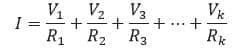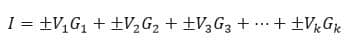3.  Now, Calculate the conductance of each current source by following a mathematical expression.4. Now, calculate the open end voltage in the parallel circuit. According to Ohm’s Law;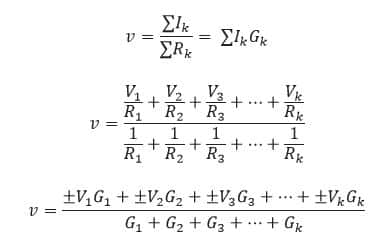5. Now find the current flowing in the load circuit RL.#### Limitations of  Millman’s Theorem

1. Applicable if the circuit has two or more independent sources.
2. Applicable if the circuit has no resistance between the independent source.
3. Applicable if the circuit has no dependent source between the independent source.

#### Applications of  Millman’s Theorem

1. This theorem is very easy for calculating the voltage across a set of parallel branches which has many voltage or current sources.
2. It is easy to apply because it does not have simultaneous equations.
3. It is mostly applied for operational amplifiers connected in the series and parallel complex circuits.

### Illustrative Examples

#### Solved Problems on Millman’s Theorem

Now we will try to understand the concept with the help of an example.

Find the voltage across 4 Ohms resistance using Millman’s theorem. Also, find the current through 4 Ohms resistance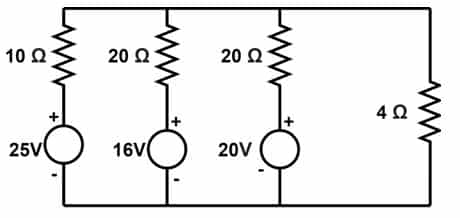Step-1

Remove the load resistance and measure the voltage at the source end.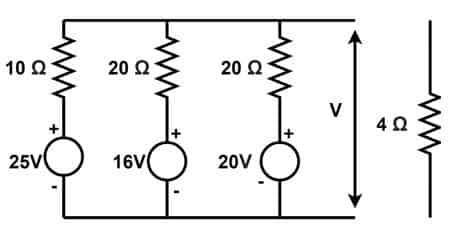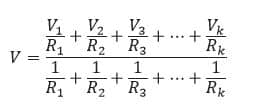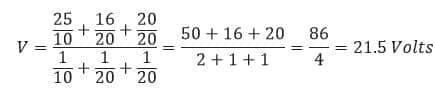The voltage across 4 Ohms resistance is 21.5 Volts.Now, the equivalent circuit is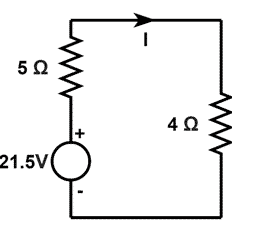Current through 4 ohms resistance is;Related Article:

1. Maximum Power Transfer Theorem
2. Kirchhoff’s Current Law, KCL and Junction Rule,Solved Problems